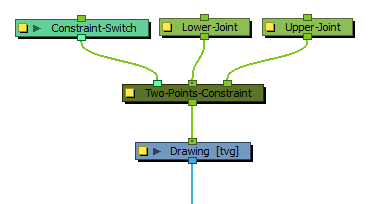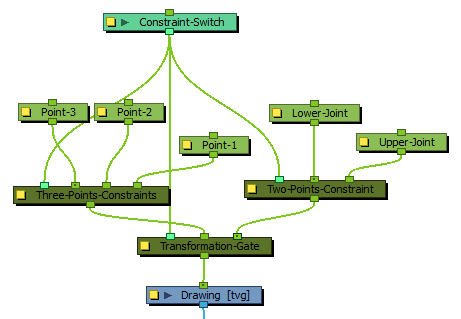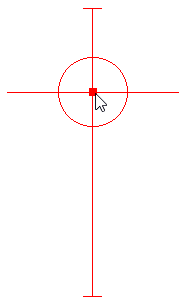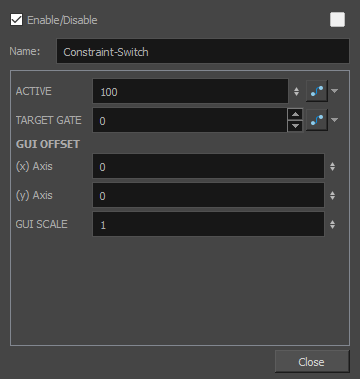# Constraint Switch Node

The Constraint Switch node is used to remotely affect the Value and/or Target Gate parameter of other Constraint nodes.

Each node in the Constraint category has an Active parameter that can be set to a value ranging from 0 to 100. This value is used to determine how much of the transformation calculated by a constraint node should actually be applied to its child element. When set to 100, the transformation is fully applied. Then set to 0, the transformation is not applied at all. When set to a value between 0 and 100, the effect the constraint node has on its child is proportionally tempered.

The Constraint Switch has its own Active parameter. When connected to a constraint node, the Active value of that constraint node is multiplied by the Active value of the Constraint Switch. For example, if a Constraint Switch with an Active parameter set to 50 is connected to a Two-Points Constraint node with an Active parameter of 50, the effective Active value of the Two-Points Constraint node will be 25, and only 25% of its transformation will be applied. Likewise, if the Active value of the Constraint Switch is set to 0, it will effectively "switch off" the constraint node, and if it is set to any value below 0, it will reverse the effect of the constraint node.

A Constraint Switch can also be used to remotely control the Target Gate parameter of a Transformation Gate. A Transformation Gate takes several transformations as inputs and outputs one of the transformations. The Target Gate is the numerical index of the port the Transformation Gate takes the transformation from when its Value parameter is set to 100. The Target Gate for a Transformation Gate can be set within its own properties, but if a Constraint Switch is connected to it, the Constraint Switch's Target Gate parameter will override the Transformation Gate's Target Gate parameter.

## Connection

The Constraint Switch node can be connected to the leftmost input port of any constraint node, except for the Static-Transformation node. The input port for a Constraint Switch will be the same shade of aquamarine as the Constraint-Switch node itself.A Constraint-Switch node can be connected to several constraint nodes to control them simultaneously.## Controls

When the control of a Transformation Switch is displayed, a slider displays in the Camera view which can be used to manually set the value of the Active parameter.## PropertiesParameter Description Active Defines the influence applied to the constraint node the Constraint Switch node is connected to. This value is multiplied with the value of the Active parameter of the constraint node to calculate its effective Active parameter. For example, if a Constraint Switch with an Active parameter set to 50 is connected to a Two-Points Constraint node with an Active parameter of 50, the effective Active value of the Two-Points Constraint node will be 25, and only 25% of its transformation will be applied. Likewise, if the Active value of the Constraint Switch is set to 0, it will effectively "switch off" the constraint node, and if it is set to any value below 0, it will reverse the effect of the constraint node. Target Gate If the Constraint Switch is connected to a Transformation Gate node, this parameter overrides the Target Gate parameter. of the Transformation Gate. GUI Offset When displaying this node's control, a slider displays in the Camera view to control the node's Active parameter. The following parameters affect the way the slider is displayed. (x) Axis Offsets the horizontal position of the slider in the Camera view.. (y) Axis Offsets the vertical position of the slider in the Camera view. GUI Scale Multiplies the size of the slider in the Camera view. This parameter is set to 1 by default.